Colors

Willie got birthday 6 colour pens in different colors. How many ways he can give them side by side in pencil?

Result

n =  720

Solution:Leave us a comment of this math problem and its solution (i.e. if it is still somewhat unclear...):Be the first to comment!Next similar math problems:

1. Committees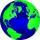How many different committees of 6 people can be formed from a class of 30 students?
2. Cards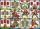How many ways can give away 32 playing cards to 5 player?
3. Big factorial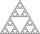How many zeros end number 116! ?
4. SickSick Marcel already taken six tablets, which was a quarter of the total number of pills in the pack. How many pills were in the pack?
5. Permutations without repetitionFrom how many elements we can create 720 permutations without repetition?
6. Theorem proveWe want to prove the sentence: If the natural number n is divisible by six, then n is divisible by three. From what assumption we started?
7. Simplify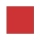Simplify powers multiplication: (3+22)(5-42)
8. MidpointsTriangle whose sides are midpoints of sides of triangle ABC has a perimeter 45. How long is perimeter of triangle ABC?
9. RoundingThe following numbers round to the thousandth:
10. The diagram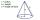The diagram is a cone of radius 8cm and height 10cm. The diameter of the base is. ..
11. Dropped sheetsThree consecutive sheets dropped from the book. The sum of the numbers on the pages of the dropped sheets is 273. What number has the last page of the dropped sheets?
12. Simple equation 9Solve the following equation: -8y+5=-9y+9
13. Fractions 3Calculate 1/9 of 27:
14. Find xSolve: if 2(x-1)=14, then x= (solve an equation with one unknown)
15. Average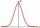Average of 7 numbers is 65. What is its sum?
16. Value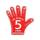Find the value of the expression: 6!·10^-3
17. Watching TVOne evening 2/3 students watch TV. Of those students, 3/8 watched a reality show. Of the students that watched the show, 1/4 of them recorded it. What fraction of the students watched and recorded reality tv.